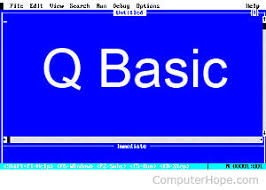How To find the area and circumference of a circle Using Qbasic - Auxiliary Tech

## Wednesday, August 21, 2019

Qbasic is a nice programming software to find the area and circumference of a circle and many other thing you can  think of....
I decided to share my knowledge to my fans and people that search about qbasic in google and any other platform......
This  is a simple coding Below that can help you Find the area and circumference of a circle.....CLS
COLOR 5
PRINT "find the area and circumference of a circle?"
LET pie = 3.14
INPUT "enter radius of circle"; r
a = pie * r ^ 2
c = 2 * pie * r
PRINT "the area of a circle is"; a
PRINT "the circumference of a circle is"; c

COLOR 7
PRINT "find the area of triangle and trapezium?"
b = 12
h = 10
area = 1 / 2 * b * h
PRINT "area of a triangle is"; area
b1 = 14
b2 = 8
area = 1 / 2 * (b1 + b2) * h
PRINT "area of a trapezium is"; area

COLOR 9
PRINT " i am done with the area and the circumference of a circle"
PRINT " and also let me explain what i just did from the top"
PRINT " i did not use dim or integer for my solution in this program"
PRINT " because i found a way that you need to do in 1min"
PRINT " just look at my program and do yours in not less than 1min"